Test: Probability- 2

# Test: Probability- 2

Test Description

## 10 Questions MCQ Test CSAT Preparation for UPSC CSE | Test: Probability- 2

Test: Probability- 2 for Quant 2023 is part of CSAT Preparation for UPSC CSE preparation. The Test: Probability- 2 questions and answers have been prepared according to the Quant exam syllabus.The Test: Probability- 2 MCQs are made for Quant 2023 Exam. Find important definitions, questions, notes, meanings, examples, exercises, MCQs and online tests for Test: Probability- 2 below.
Solutions of Test: Probability- 2 questions in English are available as part of our CSAT Preparation for UPSC CSE for Quant & Test: Probability- 2 solutions in Hindi for CSAT Preparation for UPSC CSE course. Download more important topics, notes, lectures and mock test series for Quant Exam by signing up for free. Attempt Test: Probability- 2 | 10 questions in 10 minutes | Mock test for Quant preparation | Free important questions MCQ to study CSAT Preparation for UPSC CSE for Quant Exam | Download free PDF with solutions
 1 Crore+ students have signed up on EduRev. Have you?
Test: Probability- 2 - Question 1

### What is the probability of selecting a prime number from 1,2,3,... 10 ?

Detailed Solution for Test: Probability- 2 - Question 1

Total count of numbers, n(S) = 10

Prime numbers in the given range are 2,3,5 and 7
Hence, total count of prime numbers in the given range, n(E) = 4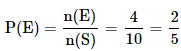Test: Probability- 2 - Question 2

### 3 balls are drawn randomly from a bag contains 3 black, 5 red and 4 blue balls. What is the probability that the balls drawn contain balls of different colors?

Detailed Solution for Test: Probability- 2 - Question 2

Total number of balls = 3 + 5 + 4 = 12

Let S be the sample space.
n(S) = Total number of ways of drawing 3 balls out of 12 = 12C3

Let E = Event of drawing 3 different coloured balls

To get 3 different coloured balls,we need to select one black ball from 3 black balls,
one red ball from 5 red balls, one blue ball from 4 blue balls

Number of ways in which this can be done = 3C1 × 5C1 × 4C1
i.e., n(E) = 3C1 × 5C1 × 4C1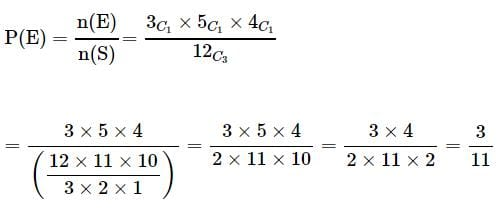Test: Probability- 2 - Question 3

### 5 coins are tossed together. What is the probability of getting exactly 2 heads?

Detailed Solution for Test: Probability- 2 - Question 3

Total number of outcomes possible when a coin is tossed = 2 (? Head or Tail)
Hence, total number of outcomes possible when 5 coins are tossed, n(S) = 25

E = Event of getting exactly 2 heads when 5 coins are tossed
n(E) = Number of ways of getting exactly 2 heads when 5 coins are tossed = 5C2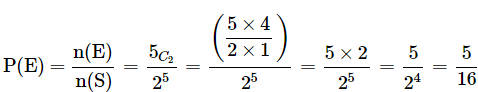Test: Probability- 2 - Question 4

What is the probability of drawing a "Queen" from a deck of 52 cards?

Detailed Solution for Test: Probability- 2 - Question 4

Total number of cards, n(S) = 52

Total number of "Queen" cards, n(E) = 4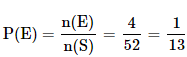Test: Probability- 2 - Question 5

A card is randomly drawn from a deck of 52 cards. What is the probability getting an Ace or King or Queen?

Detailed Solution for Test: Probability- 2 - Question 5

Total number of cards = 52

Total number of Ace cards = 4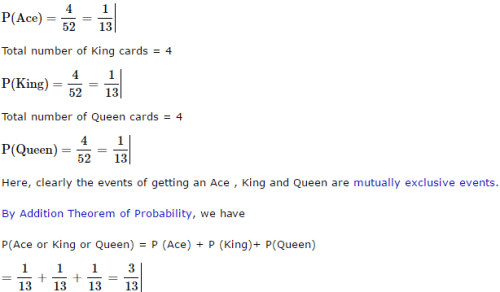Test: Probability- 2 - Question 6

A card is randomly drawn from a deck of 52 cards. What is the probability getting a five of Spade or Club?

Detailed Solution for Test: Probability- 2 - Question 6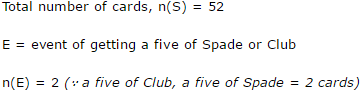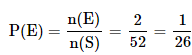Test: Probability- 2 - Question 7

When two dice are rolled, what is the probability that the sum is either 7 or 11?

Detailed Solution for Test: Probability- 2 - Question 7

Total number of outcomes possible when a die is rolled = 6 (? any one face out of the 6 faces)

Hence, total number of outcomes possible when two dice are rolled = 6 × 6 = 36

To get a sum of 7, the following are the favourable cases.
(1, 6), (2, 5), {3, 4}, (4, 3), (5, 2), (6,1)

=> Number of ways in which we get a sum of 7 = 6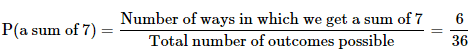To get a sum of 11, the following are the favourable cases. (5, 6), (6, 5) => Number of ways in which we get a sum of 11 = 2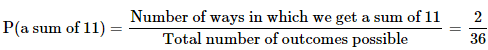Here, clearly the events are mutually exclusive events. By Addition Theorem of Probability, we have P(a sum of 7 or a sum of 11) = P(a sum of 7) + P( a sum of 11)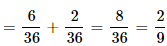Test: Probability- 2 - Question 8

A card is randomly drawn from a deck of 52 cards. What is the probability getting either a King or a Diamond?

Detailed Solution for Test: Probability- 2 - Question 8

Total number of cards = 52

Total Number of King Cards = 4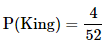Total Number of Diamond Cards = 13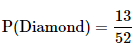Total Number of Cards which are both King and Diamond = 1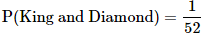Here a card can be both a Diamond card and a King. Hence these are not mutually exclusive events. (Reference : mutually exclusive events) . By Addition Theorem of Probability, we have P(King or a Diamond) = P(King) + P(Diamond) – P(King and Diamond)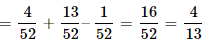Test: Probability- 2 - Question 9

John and Dani go for an interview for two vacancies. The probability for the selection of John is 1/3 and whereas the probability for the selection of Dani is 1/5. What is the probability that none of them are selected?

Detailed Solution for Test: Probability- 2 - Question 9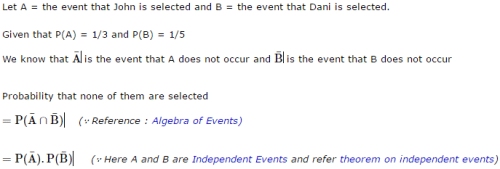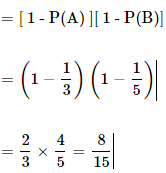Test: Probability- 2 - Question 10

John and Dani go for an interview for two vacancies. The probability for the selection of John is 1/3 and whereas the probability for the selection of Dani is 1/5. What is the probability that only one of them is selected?

Detailed Solution for Test: Probability- 2 - Question 10

The correct answer is B as
probability of one of them being selected, imply either John must be selected and Dani must not or Dani must be selected and John must not.
The probability of John's selection is 1/3, so that of his disselection is (1-1/3) = 2/3.
The probability of Dani's selection is 1/5, so that of his disselection is (1-1/5) = 4/5.
The probability of John's selection and Dani's disselection is 1/3 x 4/5 = 4/15.
The probability of Dani's selection and John's disselection is 2/3 x 1/5 = 2/15.
So the required probability is 4/15 + 2/15 = 6/15 = 2/5.

## CSAT Preparation for UPSC CSE

72 videos|69 docs|92 tests
 Use Code STAYHOME200 and get INR 200 additional OFF Use Coupon Code
Information about Test: Probability- 2 Page
In this test you can find the Exam questions for Test: Probability- 2 solved & explained in the simplest way possible. Besides giving Questions and answers for Test: Probability- 2, EduRev gives you an ample number of Online tests for practice

## CSAT Preparation for UPSC CSE

72 videos|69 docs|92 tests